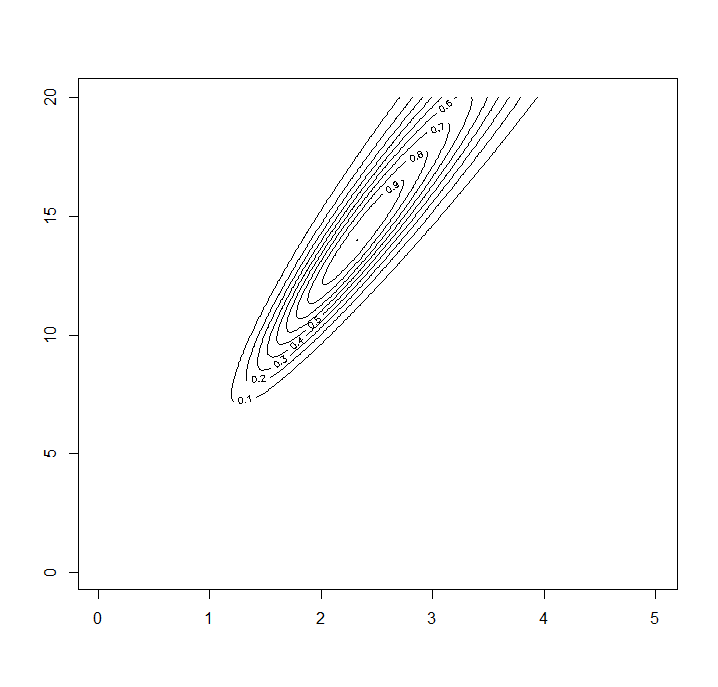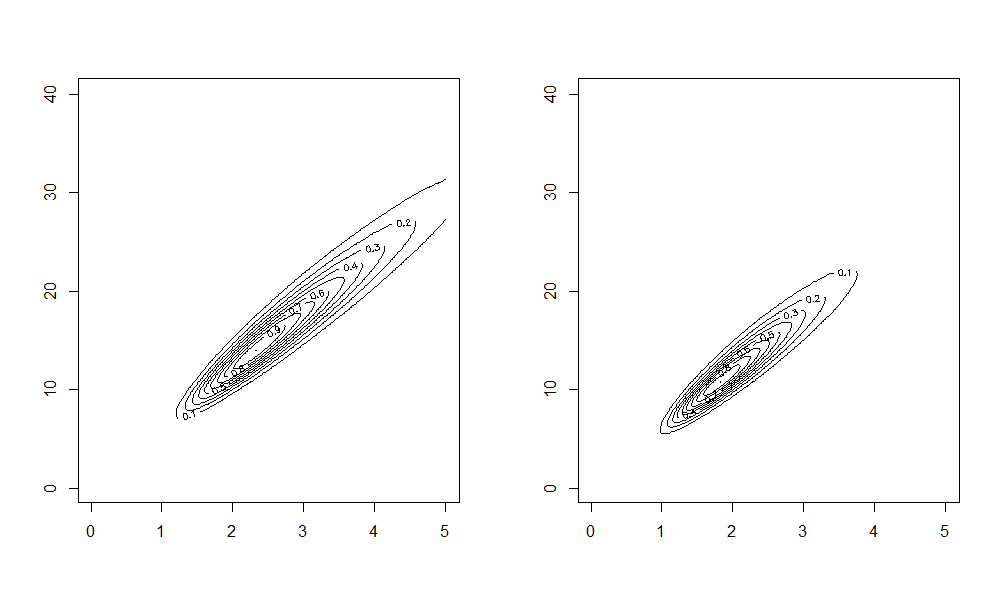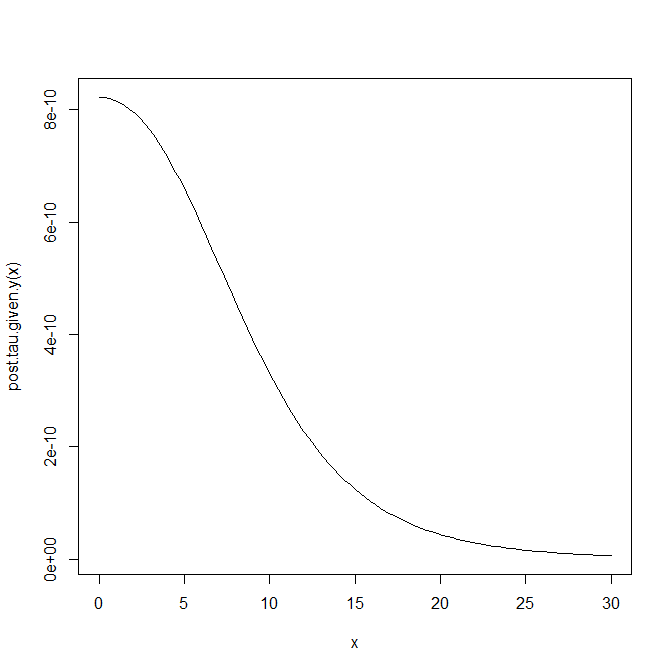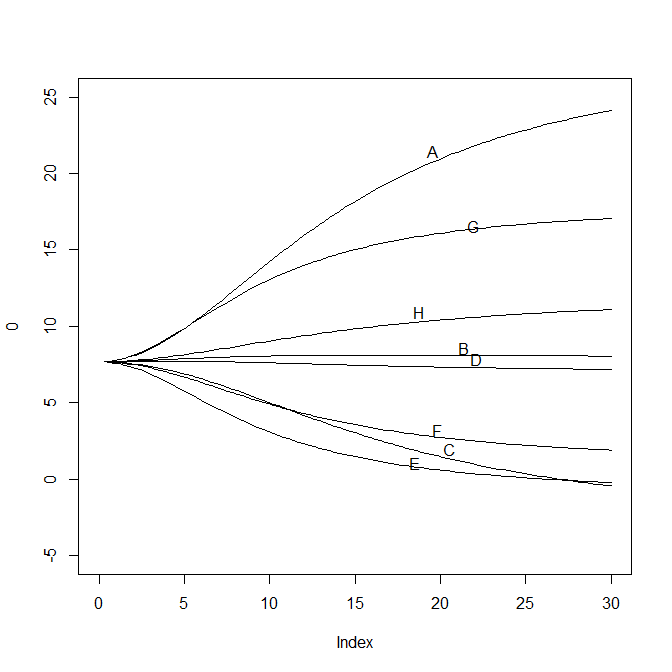## 引入

$\mathbb{E}(\theta)=\frac{\alpha}{\alpha+\beta},\,\mathrm{Var}(\theta)=\frac{\alpha\beta}{(\alpha+\beta)^2(\alpha+\beta+1)}$

$\alpha=\frac{\mathbb{E}(\theta)^2(1-\mathbb{E}(\theta))}{\mathrm{Var}(\theta)}-\mathbb{E}(\theta),\,\beta=\frac{1-\mathbb{E}(\theta)}{\mathbb{E}(\theta)}\alpha$

## 回顾

• 将模型分解为更小的子模块，每个子模块都更容易被理解，正如上面将整个模型拆成了两个易于理解的简单模型。
• 非层次化模型中，过少的参数往往难以很好的拟合模型
• 非层次化模型中，过多的参数很容易过拟合模型（因为有太多的条件概率）
• 在层次化模型中，有时甚至参数的个数可以多于数据的个数，仍能取得较合理的效果

## 框架

$y\lvert\theta,\phi\sim p(y\lvert\theta)$ $\theta\lvert\phi\sim p(\theta\lvert\phi)$ $\phi\sim p(\phi)$

$p(\theta,\phi)=p(\phi)p(\theta\lvert\phi)$ $p(\theta,\phi\lvert y)\propto p(\phi)p(\theta\lvert\phi)p(y\lvert\theta,\phi)=p(\phi)p(\theta\lvert\phi)p(y\lvert\theta)$

• 直接积分法$\int p(\theta,\phi\lvert y)\,\mathrm{d}\theta$，但积分操作在高维空间里并不容易求得。
• 条件密度法$p(\theta,\phi\lvert y)/p(\theta\lvert\phi,y)$，但分母的归一化因子通常是与$\phi$有关的，有可能其计算并不简单

## 回到最初的例子——二项分布

### 求解后验分布

$p(\alpha,\beta,\theta\lvert y)\propto p(\alpha,\beta) \prod_{J=1}^{J}\frac{\Gamma(\alpha+\beta)}{\Gamma(\alpha)\Gamma(\beta)}\theta_j^{\alpha-1}(1-\theta_j)^{\beta-1}\prod_{J=1}^{J}\theta_j^{y_j}(1-\theta_j)^{n_j-y_j}$

$\theta$的条件后验为一个$\mathrm{Beta}$分布，为

$p(\theta\lvert \alpha,\beta,y)=\prod_{J=1}^{J}\frac{\Gamma(\alpha+\beta+n_j)}{\Gamma(\alpha+y_j)\Gamma(\beta+n_j-y_j)}\theta_j^{\alpha+y_j-1}(1-\theta_j)^{\beta-1+n_j-y_j}$

$p(\alpha,\beta\lvert y)\propto p(\alpha,\beta)\prod_{J=1}^{J}\frac{\Gamma(\alpha+\beta)}{\Gamma(\alpha)\Gamma(\beta)}\frac{\Gamma(\alpha+y_j)\Gamma(\beta+n_j-y_j)}{\Gamma(\alpha+\beta+n_j)}$

### 超先验的选择

#### 为了简单## 十分重要的例子——正态分布

### 模型假设

$p(\theta,\mu,\tau\lvert y)\propto p(\mu,\tau)\prod_{j=1}^{J}\mathcal{N}(\theta_j\lvert\mu,\tau^2)\prod_{j=1}^{J}\mathcal{N}(\bar{y}_{\cdot j}\lvert\theta_j,\sigma_j^2)$

$p(\theta_j\lvert\mu,\tau,y)\sim\mathcal{N}(\widehat{\theta}_j,V_j)\qquad\widehat{\theta}_j=\frac{\frac{\bar{y}_{\cdot j}}{\sigma_j^2}+\frac{\mu}{\tau^2}}{\frac{1}{\sigma_j^2}+\frac{1}{\tau^2}},\,V_j=\frac{1}{\frac{1}{\sigma_j^2}+\frac{1}{\tau^2}}$ $p(\mu,\tau\lvert y)\propto p(\mu,\tau)\prod_{j=1}^{J}\mathcal{N}(\bar{y}_{\cdot j}\lvert\mu,\sigma_j^2+\tau^2)$

$p(\mu\lvert\tau,y)\sim\mathcal{N}(\widehat{\mu},V_\mu),\quad \widehat{\mu}=\frac{\sum_{j=1}^{J}\frac{\bar{y}_{\cdot j}}{\sigma_j^2+\tau^2}}{\sum_{j=1}^{J}\frac{1}{\sigma_j^2+\tau^2}},\,V_\mu^{-1}=\sum_{j=1}^{J}\frac{1}{\sigma_j^2+\tau^2}$

$p(\tau\lvert y)\propto\frac{p(\mu,\tau\lvert y)}{p(\mu\lvert\tau,y)}\propto \frac{p(\tau)\prod_{j=1}^J\mathcal{N}(\widehat{y}_{\cdot j}\lvert\mu,\sigma_j^2+\tau^2)}{\mathcal{N}(\mu\lvert\widehat{\mu},V_\mu)}$

$p(\tau\lvert y)\propto p(\tau)V_\mu^{1/2}\prod_{j=1}^{J}(\sigma_j^2+\tau^2)^{-1/2}\exp\left\{-\frac{(\bar{y}_{\cdot j}-\widehat{\mu})^2}{2(\sigma_j^2+\tau^2)}\right\}$

### 实际计算

1. $p(\tau\lvert y)$中抽样出$\tau_k$
2. $p(\mu\lvert \tau_k,y)$中抽样出$\mu_k$
3. $p(\theta\lvert \mu_k,\tau_k,y)$中抽样出$\theta_k$（事实上，由于$\theta_1,\ldots,\theta_J$彼此条件独立，我们这一步可以直接抽样出$J$个样本$\theta_{1,k},\ldots,\theta_{J,k}$

### 如果不用贝叶斯方法？

$\widehat{\mu}=\bar{y}_{\cdot\cdot},\,\widehat{\tau}^2=(\mathrm{MS_B}-\mathrm{MS_W})/n$

SS df MS $\mathbb{E}(\mathrm{MS}\lvert\sigma^2,\tau^2)$

## 例子分析

A 28 15
B 8 10
C -3 16
D 7 11
E -1 9
F 1 11
G 18 10
H 12 18

### 汇集预测

$\bar{y}_{\cdot\cdot}=\frac{\sum_{j=1}^{J}\bar{y}_{\cdot j}/\sigma_j^2}{\sum_{j=1}^{J}1/\sigma_j^2}=7.69$ $(\sum_{j=1}^{J}1/\sigma_j^2)^{-1/2}=4.07$

### 贝叶斯模型